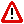To Return to the Prior Page "Click" the Browser Back Button Until the Desired Page Appears

 Triquametric motionTriquametric motion is a singular phenomenon that is at the crux of all the manifestations of Reality.

An understanding of Triquametric motion unravels the enigmas of existence.

Literally, Triquametric refers to something that radiates in all directions, simultaneously, to and from three linear points.

Triquametric motion can be said to manifest as an ellipsoid, which is comprised of complex oscillations, that has a perigee that is an integer multiple of the difference between the hypotenuse and wave.  Such ab ellipsoid is referred to as a Pulsoid, which is created from pulsating energy that is referred to as Fluence.

Triquametric motion describes the internal structure of the states of Light from which all phenomena is constructed . . . including motion, dimensions, mathematics; and even . . . thought, itself.

Triquametric motion, which is relativistic, can be, often, rather non-intuitive for many persons.  However, the motion can be reduced to a rather simple, heuristic, elliptical representation, which is the Brunardot ellipse, that is, usually, adequate for a general understanding of the complex motion.

Triquametric motion is a hyper-relativistic amalgam of solitonic resonance resulting from all the forms of oscillation: slide, swing, and vibration; and, a pulsoiding phenomenon resulting from the nature of the congruent locus of its source and terminus: Infinity.

The three points of radiation are linear with the end points at the infinite and a midpoint at the infinitesimal, which three points are, actually, a single dimensionless point until there is motion that is anywhere below that of infinite speed.  The instant there is motion a relativistic ellipsoid (Pulsoid) of almost infinite circularity manifests (The points of radiation being the foci and midpoint), which evolves to a Pulsoiding ellipsoid that is nearly a straight line; and then, morphs to a near spheroid.E-mail :  Brunardot@Brunardot.comTerms of:  ©Copyright 1999-2015 by Brunardot

000101 0:01a